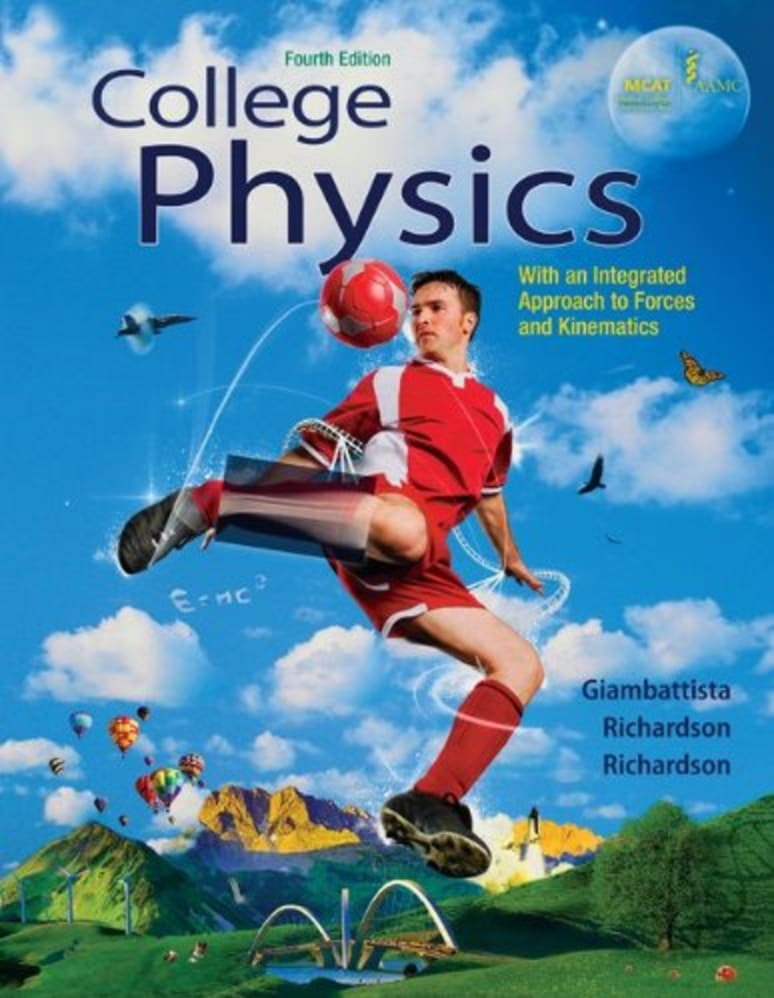Clutch Prep is now a part of Pearson
Physics College Physics# College Physics Giambattista • 4th Edition • 978-0073512143

Not the textbook you were looking for? Pick another one here.

## Ch 01: Introduction

Introduction to Units Unit Conversions Dimensional Analysis Counting Significant Figures Operations with Significant Figures

## Ch 10: Elasticity and Oscillations

Spring Force (Hooke's Law) Intro to Simple Harmonic Motion (Horizontal Springs) Energy in Simple Harmonic Motion Simple Harmonic Motion of Vertical Springs Simple Harmonic Motion of Pendulums Energy in Pendulums

## Ch 11: Waves

Intro to Waves Wave Functions & Equations of Waves Velocity of Transverse Waves (Strings) Wave Interference Standing Waves

## Ch 12: Sound

Sound Waves Standing Sound Waves Sound Intensity Beats The Doppler Effect

## Ch 13: Temperature and the Ideal Gas

Temperature Linear Thermal Expansion Volume Thermal Expansion The Ideal Gas Law Kinetic-Molecular Theory of Gases

## Ch 20: Electromagnetic Induction

Intro to Induction Magnetic Flux Faraday's Law Lenz's Law Motional EMF Transformers Mutual Inductance Self Inductance Inductors LR Circuits

## Ch 22: Electromagnetic Waves

Intro to Electromagnetic (EM) Waves The Electromagnetic Spectrum Intensity of EM Waves Wavefunctions of EM Waves Polarization & Polarization Filters

## Ch 23: Reflection and Refraction of Light

Ray Nature Of Light Law of Reflection Snell's Law Total Internal Reflection Ray Diagrams For Mirrors Mirror Equation Ray Diagrams For Lenses Thin Lens And Lens Maker Equations

## Ch 25: Interference and Diffraction

Diffraction Diffraction with Huygen's Principle Young's Double Slit Experiment Single Slit Diffraction

## Ch 26: Relativity

Inertial Reference Frames Special Vs. Galilean Relativity Consequences of Relativity Lorentz Transformations